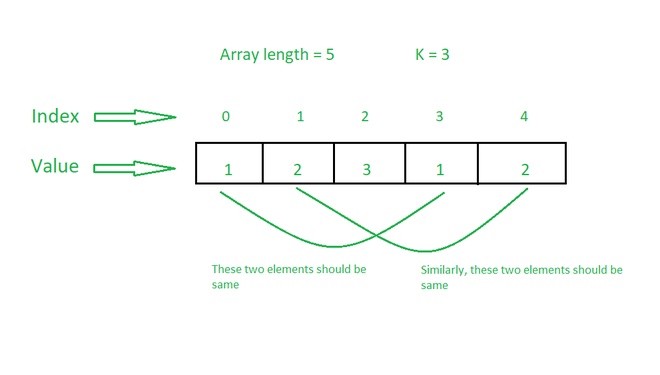Skip to content
Related Articles
Minimum replacements required to make sum of all K-length subarrays equal
• Difficulty Level : Easy
• Last Updated : 06 Jun, 2021

Given an array arr[] consisting of N positive integers and an integer K, the task is to make the sum of all K-length subarrays equal by replacing minimum number of array elements with any integer.

Examples:

Input: arr[] = {3, 4, 3, 5, 6}, K = 2
Output: 2
Explanation:
Operation 1: Replacing arr by 4 modiifes arr[] to {3, 4, 3, 4, 6}.
Operation 2: Replacing arr by 3 modifies arr[] to {3, 4, 3, 4, 3}.
All subarrays of length 2 are {{3, 4}, {4, 3}, {3, 4}, {4, 3}}. Sum of all these subarrays is 7. Therefore, the minimum number of operations required is 2.

Input: arr[] = {1, 2, 3, 1, 2}, K = 3
Output: 0
Explanation: All subarrays of length 3 are {{1, 2, 3}, {2, 3, 1}, {3, 1, 2}}. Since all these subarrays have sum 6, the number of operations required is 0.

Approach: The idea is based on the observation that all subarrays will have equal sum, when all elements separated by distance K are equal.Therefore, the problem can be solved by counting the frequency of elements separated by a distance K and find the number which appears maximum times. Follow the steps below to solve the problem:

• Initialize a variable ans to store the required result.
• Iterate in the range [0, K-1] using the variable i
• Create a map, freq to store the frequency of elements separated by a distance K starting from i.
• Traverse the map and find the element which occurs the maximum number of times.
• Again, traverse the map and if the element is not equal to the maximum occurring element then add its frequency to the ans.
• Print the value of ans as the result.

Below is the implementation of the above approach:

## C++

 `// C++ program for the above approach``#include ``using` `namespace` `std;` `// Function to find minimum number of``// operations required to make sum of``// all subarrays of size K equal``void` `findMinOperations(``int` `arr[],``                       ``int` `N, ``int` `K)``{``    ``// Stores number of operations``    ``int` `operations = 0;` `    ``// Iterate in the range [0, K-1]``    ``for` `(``int` `i = 0; i < K; i++) {` `        ``// Stores frequency of elements``        ``// separated by distance K``        ``unordered_map<``int``, ``int``> freq;` `        ``for` `(``int` `j = i; j < N; j += K)``            ``freq[arr[j]]++;` `        ``// Stores maximum frequency``        ``// and corresponding element``        ``int` `max1 = 0, num;` `        ``// Find max frequency element``        ``// and its frequency``        ``for` `(``auto` `x : freq) {``            ``if` `(x.second > max1) {``                ``max1 = x.second;``                ``num = x.first;``            ``}``        ``}` `        ``// Update the number of operations``        ``for` `(``auto` `x : freq) {``            ``if` `(x.first != num)``                ``operations += x.second;``        ``}``    ``}` `    ``// Print the result``    ``cout << operations;``}` `// Driver Code``int` `main()``{``    ``// Given Input``    ``int` `arr[] = { 3, 4, 3, 5, 6 };``    ``int` `K = 2;``    ``int` `N = ``sizeof``(arr) / ``sizeof``(arr);` `    ``// Function Call``    ``findMinOperations(arr, N, K);` `    ``return` `0;``}`

## Python3

 `# python 3 program for the above approach` `# Function to find minimum number of``# operations required to make sum of``# all subarrays of size K equal``def` `findMinOperations(arr, N, K):``    ``# Stores number of operations``    ``operations ``=` `0` `    ``# Iterate in the range [0, K-1]``    ``for` `i ``in` `range``(K):``        ``# Stores frequency of elements``        ``# separated by distance K``        ``freq ``=` `{}` `        ``for` `j ``in` `range``(i,N,K):``            ``if` `arr[j] ``in` `freq:``                ``freq[arr[j]] ``+``=` `1``            ``else``:``                ``freq[arr[j]] ``=` `1` `        ``# Stores maximum frequency``        ``# and corresponding element``        ``max1 ``=` `0``        ``num ``=` `0` `        ``# Find max frequency element``        ``# and its frequency``        ``for` `key,value ``in` `freq.items():``            ``if` `(value > max1):``                ``max1 ``=` `value``                ``num ``=` `key` `        ``# Update the number of operations``        ``for` `key,value ``in` `freq.items():``            ``if` `(key !``=` `num):``                ``operations ``+``=` `value` `    ``# Print the result``    ``print``(operations)` `# Driver Code``if` `__name__ ``=``=` `'__main__'``:``  ` `    ``# Given Input``    ``arr ``=` `[``3``, ``4``, ``3``, ``5``, ``6``]``    ``K ``=` `2``    ``N ``=` `len``(arr)` `    ``# Function Call``    ``findMinOperations(arr, N, K)``    ` `    ``# This code is contributed by ipg2016107.`

## C#

 `// C# program for the above approach``using` `System;``using` `System.Collections.Generic;` `class` `GFG{`` ` `// Function to find minimum number of``// operations required to make sum of``// all subarrays of size K equal``static` `void` `findMinOperations(``int` `[]arr,``                              ``int` `N, ``int` `K)``{``    ` `    ``// Stores number of operations``    ``int` `operations = -1;` `    ``// Iterate in the range [0, K-1]``    ``for``(``int` `i = 0; i < K; i++)``    ``{``        ` `        ``// Stores frequency of elements``        ``// separated by distance K``        ``Dictionary<``int``,``                   ``int``> freq = ``new` `Dictionary<``int``,``                                              ``int``>();``                                              ` `        ``for``(``int` `j = i; j < N; j += K)``        ``{``            ``if` `(freq.ContainsKey(arr[j]))``                ``freq[arr[j]]++;``            ``else``                ``freq.Add(arr[j], 1);``        ``}` `        ``// Stores maximum frequency``        ``// and corresponding element``        ``int` `max1 = -1, num = 0;` `        ``// Find max frequency element``        ``// and its frequency``        ``foreach``(KeyValuePair<``int``, ``int``> entry ``in` `freq)``        ``{``            ``if` `(entry.Key > max1)``            ``{``                ``max1 = entry.Value;``                ``num = entry.Key;``            ``}``        ``}``    ` `        ``// Update the number of operations``        ``foreach``(KeyValuePair<``int``, ``int``> entry ``in` `freq)``        ``{``            ``if` `(entry.Key != num)``                ``operations += entry.Value;``        ``}``    ``}` `    ``// Print the result``    ``Console.Write(operations);``}` `// Driver Code``public` `static` `void` `Main()``{``    ` `    ``// Given Input``    ``int` `[]arr = { 3, 4, 3, 5, 6 };``    ``int` `K = 2;``    ``int` `N = arr.Length;` `    ``// Function Call``    ``findMinOperations(arr, N, K);``}``}` `// This code is contributed by SURENDRA_GANGWAR`
Output:
`2`

Time Complexity: O(N)
Auxiliary Space: O(N)

Attention reader! Don’t stop learning now. Get hold of all the important DSA concepts with the DSA Self Paced Course at a student-friendly price and become industry ready.  To complete your preparation from learning a language to DS Algo and many more,  please refer Complete Interview Preparation Course.

In case you wish to attend live classes with industry experts, please refer Geeks Classes Live

My Personal Notes arrow_drop_up